Request a Free Counselling Session from our Expert Mentor# NCERT Solution for Class 10th Maths Chapter 7 Coordinate Geometry

This chapter introduces you to the fundamental concepts of coordinate geometry, including Cartesian coordinates, distance formula, and section formula.
The chapter covers various concepts related to coordinate geometry, such as plotting points on a graph, finding the distance between two points, and dividing a line segment into two parts. The solutions provided by Swastik Classes offer step-by-step explanations to all the exercises and are designed to help you understand the concepts and solve problems effectively.
Coordinate geometry is an essential part of the mathematics curriculum and is widely used in various fields such as physics, engineering, and computer science. The solutions provided by Swastik Classes will help you to build a strong foundation in coordinate geometry and prepare you for more advanced mathematical concepts.
Overall, the NCERT Solutions for Class 10th Maths Chapter 7 – Coordinate Geometry, provided by Swastik Classes, are an excellent resource for students who want to excel in the subject. The solutions will not only help them score well in their exams but also prepare them for more advanced topics in mathematics. It is crucial to have a clear understanding of the concepts covered in this chapter as it forms the foundation for more advanced concepts in coordinate geometry.

## Answers of Physics NCERT solutions for Class 10th Maths Chapter 7 Coordinate Geometry

Unit 7

Coordinate Geometry

Exercise 7.1

Question 1:

Find the distance between the following pairs of points:

(i)     (2, 3), (4, 1)

(ii)     (−5, 7), (−1, 3)

(iii)     (a, b), (− a, − b)

1. Distance between the two points is given by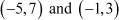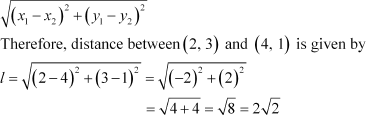1.     Distance between is given by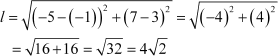1. Distance between and is given byQuestion 2:

Find the distance between the points (0, 0) and (36, 15). Can you now ﬁnd the distance between the two towns A and B discussed in Section 7.2.

Answer: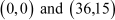Distance between points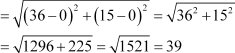Yes, we can ﬁnd the distance between the given towns A and B.

Assume town A at origin point (0, 0).

Therefore, town B will be at point (36, 15) with respect to town A.

And hence, as calculated above, the distance between town A and B will be

39 km.

Question 3:

Determine if the points (1, 5), (2, 3) and (− 2, − 11) are collinear.

Let the points (1, 5), (2, 3), and (−2, −11) be representing the vertices A, B, and C of the given triangle respectively.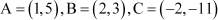Let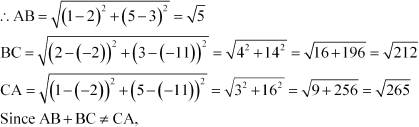Therefore, the points (1, 5), (2, 3), and (−2, −11) are not collinear.

Question 4:

Check whether (5, − 2), (6, 4) and (7, − 2) are the vertices of an isosceles triangle.

Let the points (5, −2), (6, 4), and (7, −2) are representing the vertices A, B, and C of the given triangle respectively.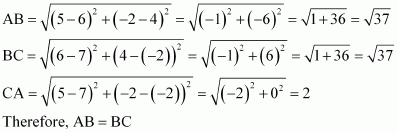As two sides are equal in length, therefore, ABC is an isosceles triangle.

Question 5: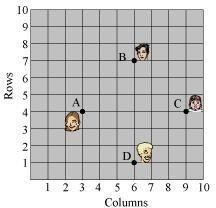In a classroom, 4 friends are seated at the points

A, B, C and D as shown in the following ﬁgure.

Champa and Chameli walk into the class and

after observing for a few minutes Champa asks

Chameli, “Don’t you think ABCD is a square?”

Chameli disagrees.

Using distance formula, ﬁnd which of them is correct.

It can be observed that A (3, 4), B (6, 7), C (9, 4), and D (6, 1) are the positions of these 4 friends.

It can be observed that all sides of this quadrilateral ABCD are of the same length and also the diagonals are of the same length.

Therefore, ABCD is a square and hence, Champa was correct.

Question 6:

Name the type of quadrilateral formed, if any, by the following points, and give reasons for your answer:

(i)  (− 1, − 2), (1, 0), (− 1, 2), (− 3, 0)

(ii) (− 3, 5), (3, 1), (0, 3), (− 1, − 4)

(iii) (4, 5), (7, 6), (4, 3), (1, 2)

1.     Let the points (−1, −2), (1, 0), (−1, 2), and (−3, 0) be representing the vertices A, B,     C, and D of the given quadrilateral respectively.

It can be observed that all sides of this quadrilateral are of the same length and also, the diagonals are of the same length. Therefore, the given points are the vertices of a square.

1. Let the points (− 3, 5), (3, 1), (0, 3), and (−1, −4) be representing the vertices A, B,     C, and D of the given quadrilateral respectively.

It can be observed that all sides of this quadrilateral are of different lengths. Therefore, it can be said that it is only a general quadrilateral, and not speciﬁc such as square, rectangle, etc.

1. Let the points (4, 5), (7, 6), (4, 3), and (1, 2) be representing the vertices A, B, C,     and D of the given quadrilateral respectively.

It can be observed that opposite sides of this quadrilateral are of the same length. However, the diagonals are of different lengths. Therefore, the given points are the vertices of a parallelogram.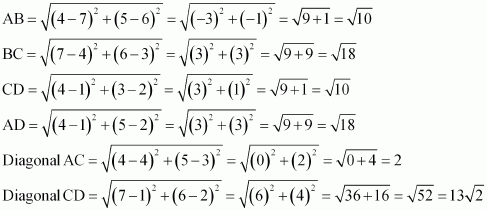*Question 7:

Find the point on the x-axis which is equidistant from (2, − 5) and (− 2, 9).

We have to ﬁnd a point on x-axis. Therefore, its y-coordinate will be 0.

Let the point on x-axis be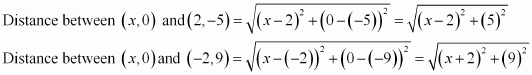By the given condition, these distances are equal in measure.

Therefore, the point is (− 7, 0).

*Question 8:

Find the values of y for which the distance between the points P (2, − 3) and Q (10, y) is 10 units.

It is given that the distance between (2, −3) and (10, y) is 10.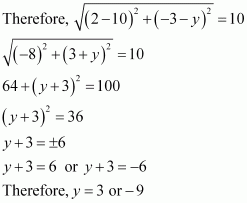*Question 9:

If Q (0, 1) is equidistant from P (5, − 3) and R (x, 6), ﬁnd the values of x. Also, ﬁnd the distance QR and PR.

Therefore, point R is (4, 6) or (−4, 6).

When point R is (4, 6),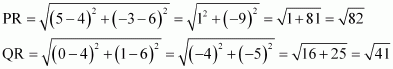When point R is (−4, 6),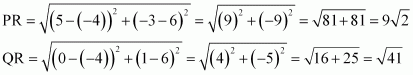*Question 10:

Find a relation between x and y such that the point (x, y) is equidistant from the point (3, 6) and (− 3, 4).

Point (x, y) is equidistant from (3, 6) and (−3, 4).

x-32+y-62=x+32+y-42

9-6x+36-12y=9+6x+16-8y

12x+4y=20

3x+y=5

Hence the relation between x and y can be given by: 3x+y=5

Exercise 7.2

Question1:

Find the coordinates of the point which divides the join of (− 1, 7) and (4, − 3) in the ratio 2:3.

Let P(x, y) be the required point. Using the section formula, we obtain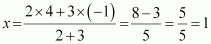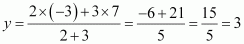Therefore, the point is (1, 3).

Question 2:

Find the coordinates of the points of trisection of the line segment joining (4, − 1) and (− 2, − 3).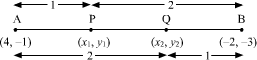Let P (x1, y1) and Q (x2, y2) are the points of trisection of the line segment joining the given points i.e., AP = PQ = QB

Therefore, point P divides AB internally in the ratio 1:2.

Point Q divides AB internally in the ratio 2:1.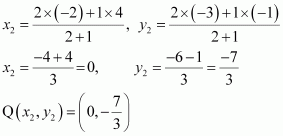Question 3:

To conduct Sports Day activities, in your rectangular shaped school ground ABCD, lines have been drawn with chalk powder at a distance of 1 m each. 100 ﬂower pots have been placed at a distance of 1 m from each other along AD, as shown in the following

ﬁgure. Niharika runs the distance AD on the 2nd line and posts a green ﬂag. Preet runs the distance AD on the eighth line and posts a red ﬂag. What is the distance between both the ﬂags? If Rashmi has to post a blue ﬂag exactly halfway between the line segment joining the two ﬂags, where should she post her ﬂag?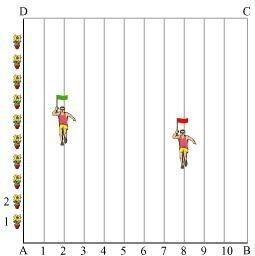It can be observed that Niharika posted the green ﬂag atof the distance AD i.e., point G is (2, 25). from the starting point of 2nd line. Therefore, the coordinates of this

Similarly, Preet posted red ﬂag atof the distance AD i.e.,    m from the starting point of 8th line. Therefore, the coordinates of this point R are (8, 20).

Distance between these ﬂags by using distance formula = GR

=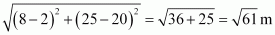The point at which Rashmi should post her blue ﬂag is the mid-point of the line joining these points. Let this point be A (x, y).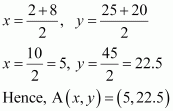Therefore, Rashmi should post her blue ﬂag at 22.5m on 5th line.

Question 4:

Find the ratio in which the line segment joining the points (− 3, 10) and (6, − 8) is divided by (− 1, 6).

Let the ratio in which the line segment joining (−3, 10) and (6, −8) is divided by point (−1, 6) be k:1.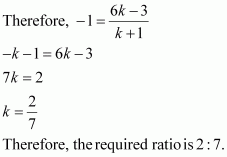Question 5:

Find the ratio in which the line segment joining A (1, − 5) and B (− 4, 5) is divided by the x-axis. Also, ﬁnd the coordinates of the point of division.

Let the ratio in which the line segment joining A (1, −5) and B (−4, 5) is divided by x-axis bek : 1

Therefore, the coordinates of the point of division is

We know that y-coordinate of any point on x-axis is 0.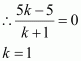Therefore, x-axis divides it in the ratio 1:1.

Division point

Question 6:

If (1, 2), (4, y), (x, 6) and (3, 5) are the vertices of a parallelogram taken in order, ﬁnd x and y.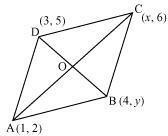Let (1, 2), (4, y), (x, 6), and (3, 5) are the coordinates of A, B, C, D vertices of a parallelogram ABCD. Intersection point O of diagonal AC and BD also divides these diagonals.

Therefore, O is the mid-point of AC and BD.

If O is the mid-point of AC, then the coordinates of O are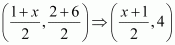If O is the mid-point of BD, then the coordinates of O are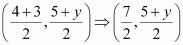Since both the coordinates are of the same point O,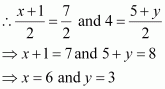*Question 7:

Find the coordinates of a point A, where AB is the diameter of circle whose centre is  (2, −3) and B is (1, 4)

Let the coordinates of point A be (x, y).

Mid-point of AB is (2, −3), which is the center of the circle.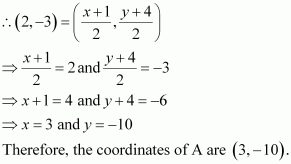*Question 8:

If A and B are (− 2, − 2) and (2, − 4), respectively, ﬁnd the coordinates of P such that and P lies on the line segment AB.The coordinates of point A and B are (−2, −2) and (2, −4) respectively.

Since

Therefore, AP: PB = 3:4

Point P divides the line segment AB in the ratio 3:4.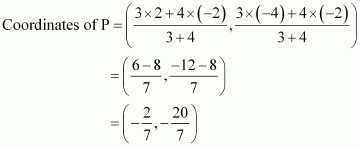*Question 9:

Find the coordinates of the points which divide the line segment joining A (− 2, 2) and B (2, 8) into four equal parts.

Answer: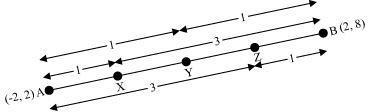From the ﬁgure, it can be observed that points P, Q, R are dividing the line segment in a ratio 1:3, 1:1, 3:1 respectively.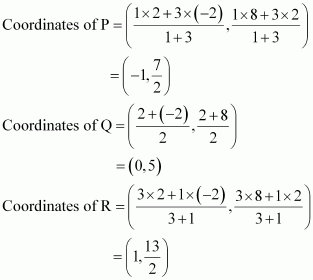*Question 10:

Find the area of a rhombus if its vertices are (3, 0), (4, 5), (− 1, 4) and (− 2, −1) taken in order. [Hint: Area of a rhombus (product of its diagonals)]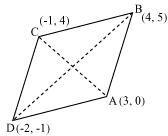Let (3, 0), (4, 5), (−1, 4) and (−2, −1) are the vertices A, B, C, D of a rhombus ABCD.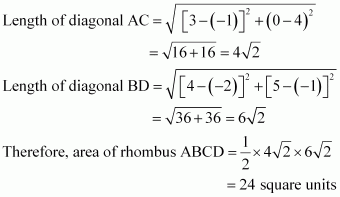Exercise 7.3

Question 1:

Find the area of the triangle whose vertices are:

(i)     (2, 3), (− 1, 0), (2, − 4)

(ii)     (− 5, −1), (3, − 5), (5, 2)

(i)     Area of a triangle is given by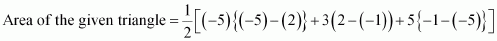(ii)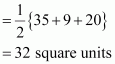Question 2:

Question 2:

In each of the following ﬁnd the value of ‘k’, for which the points     are collinear.

(i)    (7, − 2), (5, 1), (3, − k)

(ii)     (8, 1), (k, − 4), (2, − 5)

1.         For collinear points, the area of the triangle formed by them is zero.

Therefore, for points (7, −2) (5, 1), and (3, k), area = 0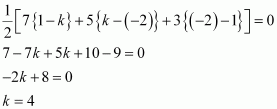1.         For collinear points, area of triangle formed by them is zero.

Therefore, for points (8, 1), (k, −4), and (2, −5), area = 0

Question 3:

Find the area of the triangle formed by joining the mid-points of the sides of the triangle whose vertices are (0, − 1), (2, 1) and (0, 3). Find the ratio of this area to the area of the given triangle.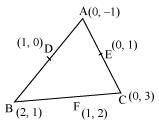Let the vertices of the triangle be A (0, −1), B (2, 1), C (0, 3).

Let D, E, F be the mid-points of the sides of this triangle. Coordinates of D, E, and F are given by

*Question 4:

Find the area of the quadrilateral whose vertices, taken in order, are (− 4, − 2), (− 3, − 5), (3, − 2) and (2, 3)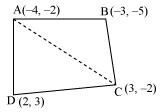Let the vertices of the quadrilateral be A (−4, −2), B (−3, −5), C (3, −2), and D (2, 3). Join AC to form two triangles ΔABC and ΔACD.

*Question 5:

You have studied in Class IX that a median of a triangle divides it into two triangles of equal areas. Verify this result for ΔABC whose vertices are A (4, − 6), B (3, − 2) and C (5, 2)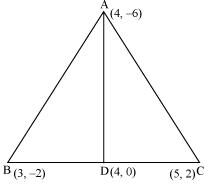Let the vertices of the triangle be A (4, −6), B (3, −2), and C (5, 2).

Let D be the mid-point of side BC of ΔABC. Therefore, AD is the median in ΔABC.

However, area cannot be negative. Therefore, area of ΔABD is 3 square units.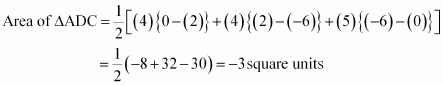However, area cannot be negative. Therefore, area of ΔADC is 3 square units.

Clearly, median AD has divided ΔABC in two triangles of equal areas.

Exercise 7.4

*Question 1:

Determine the ratio in which the line 2x + y − 4 = 0 divides the line segment joining the points A(2, − 2) and B(3, 7)

Let the given line divide the line segment joining the points A(2, −2) and B(3, 7) in a ratio k : 1.

Coordinates of the point of division

This point also lies on 2x + y − 4 = 0

Therefore, the ratio in which the line 2x + y − 4 = 0 divides the line segment joining the points A(2, −2) and B(3, 7) is 2:9.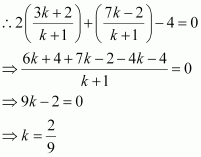*Question 2:

Find a relation between x and y if the points (x, y), (1, 2) and (7, 0) are collinear.

If the given points are collinear, then the area of triangle formed by these points will be 0.

This is the required relation between x and y.

*Question 3:

Find the centre of a circle passing through the points (6, − 6), (3, − 7) and (3, 3).

Let O (x, y) be the centre of the circle. And let the points (6, −6), (3, −7), and (3, 3) be representing the points A, B, and C on the circumference of the circle.

On adding equation (1) and (2), we obtain

From equation (1), we obtain

Therefore, the centre of the circle is (3, −2).

*Question 4:

The two opposite vertices of a square are (− 1, 2) and (3, 2). Find the coordinates of the other two vertices.

Let ABCD be a square having (−1, 2) and (3, 2) as vertices A and C respectively. Let (x, y), (x1, y1) be the coordinate of vertex B and D respectively.

We know that the sides of a square are equal to each other.

∴ AB = BC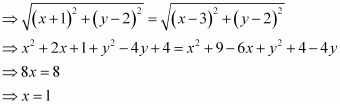We know that in a square, all interior angles are of 90°.

In ΔABC,

or 4

We know that in a square, the diagonals are of equal length and bisect each other at 90°. Let O be the mid-point of AC. Therefore, it will also be the mid-point of BD.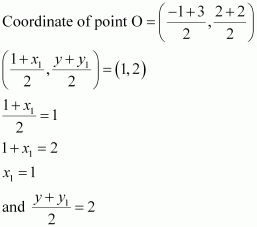If

If

Therefore, the required coordinates are (1, 0) and (1, 4).

*Question 5:

The class X students of a secondary school in Krishinagar have been allotted a rectangular plot of land for their gardening activity. Saplings of Gulmohar are planted on the boundary at a distance of 1 m from each other. There is a triangular grassy lawn in the plot as shown in the following ﬁgure. The students are to sow seeds of ﬂowering plants on the remaining area of the plot.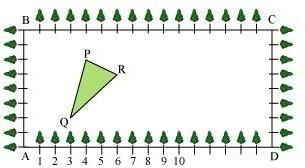1.  Taking A as origin, ﬁnd the coordinates of the vertices of the triangle.
2.     What will be the coordinates of the vertices of Δ PQR if C is the origin? Also calculate the areas of the triangles in these cases. What do you observe?

(i)        Taking A as origin, we will take AD as x-axis and AB as y-axis. It can be             observed that the coordinates of point P, Q, and R are (4, 6), (3, 2), and (6,         5) respectively.

(ii)        Taking C as origin, CB as x-axis, and CD as y-axis, the coordinates of vertices         P, Q, and R are (12, 2), (13, 6), and (10, 3) respectively.

It can be observed that the area of the triangle is same in both the cases.

*Question 6:

The vertices of a ΔABC are A (4, 6), B (1, 5) and C (7, 2). A line is drawn to intersect

sides AB and AC at D and E respectively, such that Calculate the area of the ΔADE and compare it with the area of ΔABC. (Recall Converse of basic

proportionality theorem and Theorem 6.6 related to ratio of areas of two similar triangles)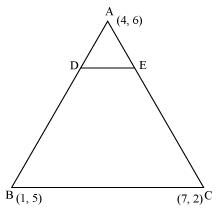Given that,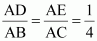Therefore, D and E are two points on side AB and AC respectively such that they divide side AB and AC in a ratio of 1:3.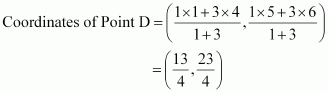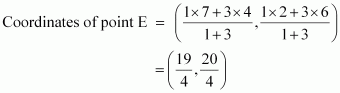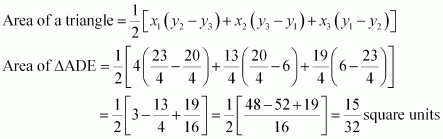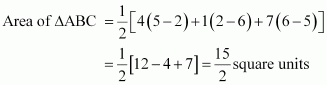Clearly, the ratio between the areas of ΔADE and ΔABC is 1:16.

Alternatively,

We know that if a line segment in a triangle divides its two sides in the same ratio, then the line segment is parallel to the third side of the triangle. These two triangles so formed (here ΔADE and ΔABC) will be similar to each other.

Hence, the ratio between the areas of these two triangles will be the square of the ratio between the sides of these two triangles.

Therefore, ratio between the areas of ΔADE and ΔABC

*Question 7:

Let A (4, 2), B (6, 5) and C (1, 4) be the vertices of ΔABC.

1.         The median from A meets BC at D. Find the coordinates of point D.
1.         Find the coordinates of the point P on AD such that AP: PD = 2:1
1.     Find the coordinates of point Q and R on medians BE and CF respectively         such that BQ: QE = 2:1 and CR: RF = 2:1.
2.     What do you observe?
1.         If A(x1, y1), B(x2, y2), and C(x3, y3) are the vertices of ΔABC, ﬁnd the coordinates         of the centroid of the triangle.1.         Median AD of the triangle will divide the side BC in two equal parts.

Therefore, D is the mid-point of side BC.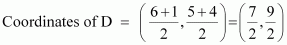1.         Point P divides the side AD in a ratio 2:1.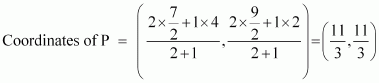1.     Median BE of the triangle will divide the side AC in two equal parts.

Therefore, E is the midpoint of side AC.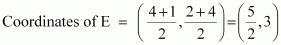Point Q divides the side BE in a ratio 2:1.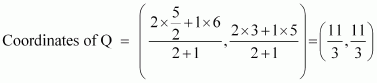Median CF of the triangle will divide the side AB in two equal parts. Therefore, F is the mid-point of side AB.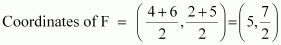Point R divides the side CF in a ratio 2:1.

1.     It can be observed that the coordinates of point P, Q, R are the same.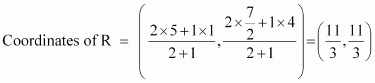Therefore, all these are representing the same point on the plane i.e., the     centroid of the triangle.

1.         Consider a triangle, ΔABC, having its vertices as A(x1, y1), B(x2, y2), and             C(x3,y3).

Median AD of the triangle will divide the side BC in two equal parts. Therefore,     D is the mid-point of side BC.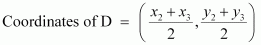Let the centroid of this triangle be O.

Point O divides the side AD in a ratio 2:1.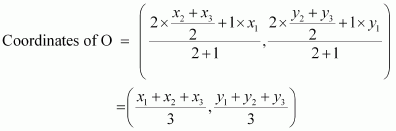*Question 8:

ABCD is a rectangle formed by the points A (− 1, − 1), B (− 1, 4), C (5, 4) and D (5, − 1). P, Q, R and S are the mid-points of AB, BC, CD, and DA respectively. Is the quadrilateral PQRS is a square? a rectangle? or a rhombus? Justify your answer.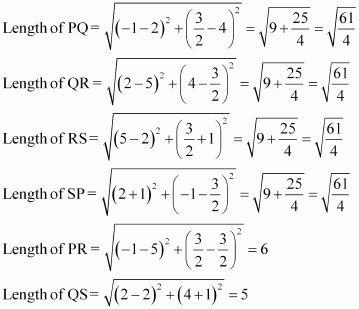It can be observed that all sides of the given quadrilateral are of the same measure. However, the diagonals are of different lengths. Therefore, PQRS is a rhombus.

This chapter comes under unit 6 and has a weightage of 6 marks in the board examination. There will be one mark MCQ question, 2mark reasoning questions, and 3 marks short answer questions. This chapter has fundamental concepts that lay the foundation for your future studies.

### Sub-topics of class 10 chapter 7 Coordinate Geometry

• Introduction to Coordinate Geometry
• Distance Formula
• Section Formula
• Area of the Triangle

### List of Exercise from Class 10 Maths Chapter 7 Coordinate Geometry

Exercise 7.1– 10 Questions which includes 8 practical based questions, 2 reasoning questions
Exercise 7.2– 10 Questions which includes 8 long answer questions, 2 short answer questions
Exercise 7.3– 5 Questions which includes 3 long answer questions, 2 practical based questions
Exercise 7.4– 8 Questions which includes 6 long answer questions, 1 practical based question, 1 reasoning question   NCERT Solutions are carefully drafted to assist the student in scoring good marks in the examination. It provides you with much-needed problem-solving practise.

This chapter deals with finding the area between two points whose coordinate values are provided. For instance the area of a triangle. This chapter has some basic concepts like the area of a triangle, rhombus, the distance between sides, and intersections. This chapter teaches you the relationship between numerical and geometry and their application in our daily lives.

These NCERT Solutions for Class 10 Maths have different types of questions and their answers which will help you with alternate solutions, diagrammatic representation. The solutions provided here are written in simple language with apt information. By studying these NCERT solutions thoroughly students will be able to solve complex problems easily.

### Key Features of NCERT Solutions for Class 10 Maths Chapter 7 Coordinate Geometry

• The solution has all the exercise questions provided in the NCERT textbook.
• Diagrammatic representations and alternate methods will help you understand the concepts thoroughly.
• Solving these NCERT Solutions will make you acquainted with important formulas and standards.
• This NCERT Solution has different examples that will help you relate to real-life examples to relate geometry and numerical.

Conclusion

The NCERT Solutions for Class 10th Maths Chapter 7 – Coordinate Geometry, provided by Swastik Classes, offer a comprehensive understanding of the fundamental concepts of coordinate geometry. The chapter covers various topics such as plotting points on a graph, finding the distance between two points, and dividing a line segment into two parts.
The solutions provided by Swastik Classes are well-structured and easy to understand, with step-by-step explanations for all the exercises. The solutions are designed to help students develop their problem-solving skills and gain a deeper understanding of the concepts covered in the chapter.
Coordinate geometry is an essential part of the mathematics curriculum and is widely used in various fields such as physics, engineering, and computer science. The solutions provided by Swastik Classes will help students build a strong foundation in coordinate geometry and prepare them for more advanced mathematical concepts.
Overall, the NCERT Solutions for Class 10th Maths Chapter 7 – Coordinate Geometry, provided by Swastik Classes, are an excellent resource for students who want to excel in the subject. The solutions will not only help them score well in their exams but also prepare them for more advanced topics in mathematics. It is crucial to have a clear understanding of the concepts covered in this chapter as it forms the foundation for more advanced concepts in coordinate geometry.

### How NCERT Solutions for Class 10 Maths Chapter 7 helpful for board exams?

NCERT Solutions for Class 10 Maths Chapter 7 provides answers with detailed descriptions as per term limit particularised by the Board for self-evaluation. Solving these solutions will provide excellent practice for the students so they can finish the paper on time. So, it’s clear that the NCERT Solutions for Class 10 Maths Chapter 7 are essential to score high in examinations. Students can get acquainted with writing exams and will be able to face exams more confidently.

### Mention the topics that are covered in NCERT Solutions for Class 10 Maths Chapter 7?

The topics that are covered in NCERT Solutions for Class 10 Maths Chapter 7 are introduction to coordinate geometry, distance Formula, section formula and area of the triangle. By learning these NCERT solutions thoroughly students will be able to solve complex problems easily.

### Is it necessary to practice all the exercises present in Chapter 7 of NCERT Solutions for Class 10 Maths?

Yes, it is compulsory to practice all the exercises present in Chapter 7 of NCERT Solutions for Class 10 Maths. Because all exercises contain numerous questions to solve which may come in their finals. This makes them more confident towards the syllabus they have.